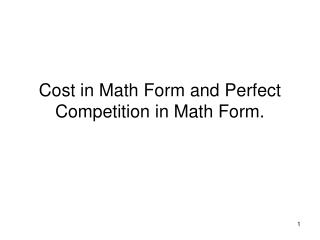DownloadDownload PresentationCost in Math Form and Perfect Competition in Math Form.

# Cost in Math Form and Perfect Competition in Math Form.

Télécharger la présentation## Cost in Math Form and Perfect Competition in Math Form.

- - - - - - - - - - - - - - - - - - - - - - - - - - - E N D - - - - - - - - - - - - - - - - - - - - - - - - - - -
##### Presentation Transcript

1. Cost in Math Form and Perfect Competition in Math Form.

2. Say we have a cost function of the general form TC = f + aQ + bQ^2 + dQ^3, where the sign ^ means raise to a power. Note the term f has no Q “hooked” on to it so f represents fixed cost, and all the terms with a Q are part of variable cost: TFC = f = total fixed cost TVC = aQ + bQ^2 + dQ^3 = total variable cost. Now, it is possible that a, b, and d are positive or negative, or even 0. Looking at averages we have AFC = f/Q, AVC = (aQ + bQ^2 + dQ^3)/Q = a + bQ + dQ^2, And ATC = AFC + AVC = TC/Q.

3. To get the marginal cost from a TC of the form TC = f + aQ + bQ^2 + dQ^3, you have MC = a + 2bQ + 3dQ^2. Example 1 TC = 100 + Q^2 Note f = 100 and a=0, b=a, and d =0. AFC = 100/Q, AVC = Q and MC = 2Q. Say this firm operates in a perfectly competitive market where the price is \$10. From my notes the production rule says go to the quantity where P = MC, so 10 = 2Q, or Q = 5. The operating rule says only do this if P > AVC. Here AVC = Q and at Q = 5 AVC = 5 and thus P is > AVC. SO the best thing to do is make Q=5.

4. Profit = TR – TC = PQ – [TC/Q]Q = [P – ATC]Q Here I used a trick that TC times 1 written as Q/Q gives [TC/Q]Q = ATCQ. Thus, profit = [P – ATC]Q. In our problem we have [10 – 25]5 = -75. So, the best this firm can do is lose 75. But, note if it shut down it would still have fixed cost of 100 that would have to be paid. So, it is better off operating in the short run while it has the 100 fixed cost. Example 2 – same cost, but say the price in the market is 20. (note this problem is not exactly like demo problem 8-1) Produce Q where P = MC: 20 = 2Q, or Q = 10. Make sure P > AVC: AVC here is 10, so with P > AVC operate. Profit = [20 – 20]10 = 0.